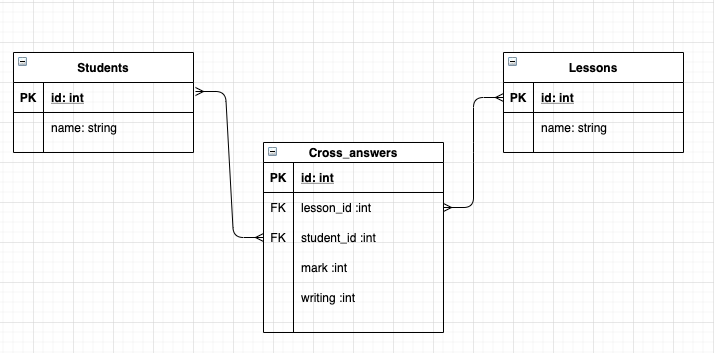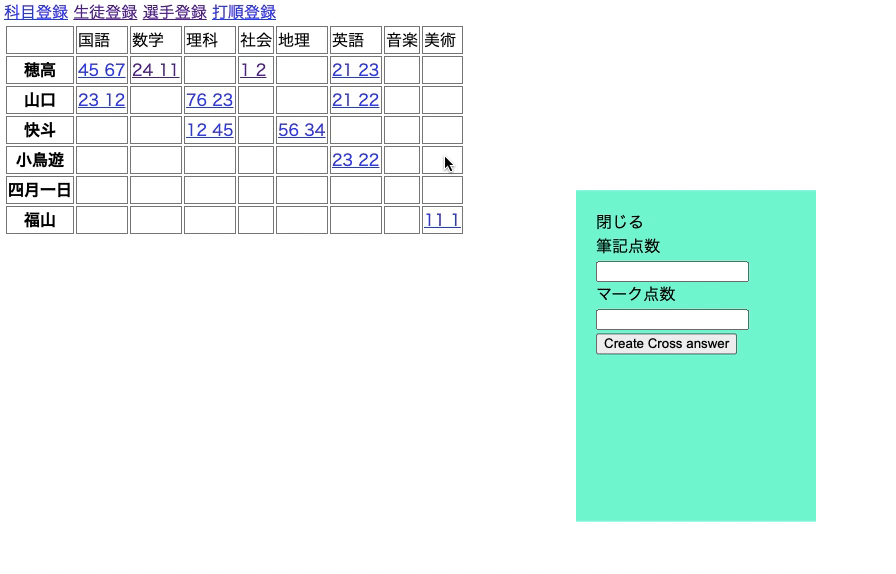# Background of creation

Currently, we are creating an app that records baseball scorebooks. If you want to register the results of the first and first at-bats of baseball I decided to create a mini app and check the behavior, thinking that there is a function that can display the registration form by clicking the place where 1 at bat and 1 at bat cross in the scorebook.

# Mini app specifications

We have created a matrix table that shows how many points students have scored in which subjects. You can enter the score by clicking the crossed part of the table. Also, for the items that have already been entered, the input values ​​can be updated.

### DB design### Completion image diagram# How to make

1. Create a routing
2. Creating a controller
3. Create view
4. Creating Javascript

### 1. Create a routing

#### `routes.rb`

``````
Rails.application.routes.draw do
resources :students, only: [:index, :new, :create]
resources :lessons, only: [:index, :new, :create]
resources :cross_answers, only: [:index, :create, :update, :destroy]
end
``````

### 2. Creating a controller

#### `cross_answers_controller.rb`

``````
def index
@lessons = Lesson.all
@students = Student.all
If the parameter is displayed, the data of the specified cross answer is displayed.
if params[:lesson_id] && params[:student_id]
else
end

end
def create
redirect_to root_path
else
render :index
end
end

def update
redirect_to root_path
else
render :index
end
end

private
end

end

end
``````

#### Supplement

It should be noted that the process is branched from the case where the parameter is sent at the time of the index action. When there is no parameter, the value stored in the instance variable is changed in order to execute the registration and the update process in some cases. At the time of update, student_id and lesson_id are sent as parameters, and unique data that matches the ID is stored in a variable and used in the view.

### 3. Create view

#### `cross_answers/index.html`

``````
<div id="cross-matrix">
<table class="matrix">
//Display subject names in the matrix table at the top
<tr class="matrix">
<td class="matrix"></td>
<% @lessons.each do |lesson| %>
<td class="matrix">
<%= lesson.name %>
</td>
<% end %>
</tr>
<tbody>
//Output items for student names
<% @students.each do |student| %>
<tr class="matrix">
//Display student name on the left
<th class="matrix">
<%= student.name %>
</th>
//Display subject item sequence
<% @lessons.each do |lesson| %>
//Stores hidden student table and subject table IDs
<div class="hidden lesson-num">
<%= lesson.id%>
</div>
<div class="hidden student-num">
<%= student.id %>
</div>
//If there is a cross answer table with student ID and subject ID, two test scores will be displayed.
<% if @cross_answers.find_by(lesson_id: lesson.id, student_id: student.id).present? %>
//When the score is displayed, launch the edit form instead of the registration form.
<% end %>
<% end %>
</td>
<% end %>
</tr>
<% end %>
</tbody>
</table>
</div>
//If the parameters do not have a student ID and subject ID, click the cross section to launch the registration form.
<% unless params[:lesson_id] && params[:student_id] %>
<section id="modal" class="hidden">
<div id="close">
close
</div>
<%= form_with model: @cross_answer, local: true do |f| %>
<%= f.text_field :lesson_id, class:"hidden",id:"lesson_id-input"%>
<%= f.text_field :student_id, class:"hidden", id:"student_id-input" %>
<div>
<label>Written score</label>
<%= f.text_field :writing %>
</div>
<div>
<label>Mark score</label>
<%= f.text_field :mark %>
</div>
<%= f.submit %>
<% end %>
</section>
</div>
<% else %>
//If the parameters include student ID and subject ID, the edit form will remain displayed.
<section id="modal">
//Click Close to move to the page without cross answer parameters
<div id="edit-close">
close
</div>
<% end %>
<%= f.hidden_field :id %>
<%= f.text_field :lesson_id, class:"hidden",id:"lesson_id-input"%>
<%= f.text_field :student_id, class:"hidden", id:"student_id-input" %>
<div>
<label>Written score</label>
<%= f.text_field :writing %>
</div>
<div>
<label>Mark score</label>
<%= f.text_field :mark %>
</div>
<%= f.submit %>
<% end %>
</section>
</div>
<% end %>
``````

#### Supplement

__ How to make a matrix table __ We are creating a table with the table tag. The first line uses the each method to increase the subject name sequence and display the subject name. From the second line onward, use the each method to increase the number of student name lines. The student name is displayed in the first column, and the subsequent columns use the each method again to increase the subject name segment. It has a nested structure in which each method is stored in each method. Now you can create a matrix table.

__ About the stored data in the table __ The table can store 4 data. ・ Hidden subject ID ・ Hidden student ID If there is data in the cross answer table with the above two IDs, it will be displayed. ・ Mark score ・ Writing points

When you click the crossed place, copy the student ID and subject ID in the table and paste it in the text box for entering the hidden subject ID and student ID on the registration form.

__ About switching between registration and update __ If there is no data, click the blank part of the matrix to display the registration form with JavaScript If there is data, click the numerical value to send the parameters of student ID and subject ID to the controller, store the unique cross-answer data that matches the two IDs as an instance variable, and return it to the client. Also, in the view settings, if there are parameters, the update form will always be launched.

__ About class name __ A common class name is given to all crossing points between subjects and students in the matrix table. This is so that the event can be fired at the clicked point in JavaScript.

__ About ID name __ Give an ID name to the HTML element you want to use in JavaScript.

### 4. Creating Javascript

#### `modal.js`

``````
//Get all elements because the HTML element that inputs the form has a common class name
let info_num = cross_info.length;
//Make sure that the event occurs where you click.
for(let i=0; i<=info_num - 1; i++){
cross_info[i].onclick = function(){
//Obtain the student ID and subject ID and post them to the input items on the registration form.
let lessonId = this.children.innerHTML.trim();
let studentId = this.children.innerHTML.trim();
const modal = document.getElementById("modal");
const close = document.getElementById("close");
const studentInput = document.getElementById("student_id-input");
const lessonInput = document.getElementById("lesson_id-input");
studentInput.value = Math.floor(studentId);
lessonInput.value = Math.floor(lessonId);
//Remove hidden class names
modal.classList.remove("hidden");
//When you press close, add the hidden class name
close.onclick = function(){
};
}
}
}